Most Affordable JEE | NEET | 8,9,10 Preparation by Kota's Top IITian Doctor Faculties

# NCERT Solutions for Class 9 Maths chapter 2 Exercise 2.5 - Polynomials - Free PDF Download

Class 9Hey, are you a class 9 Student and Looking for Ways to Download NCERT Solutions for Class 9 Maths chapter 2 Exercise 2.5? If Yes then you are at the right place.

Here we have listed Class 9 maths chapter 2 exercise 2.5 solutions in PDF that is prepared by Kota’s top IITian’s Faculties by keeping Simplicity in mind.

If you want to score high in your class 9 Maths Exam then it is very important for you to have a good knowledge of all the important topics, so to learn and practice those topics you can use eSaral NCERT Solutions.

So, without wasting more time Let’s start.

### Download The PDF of NCERT Solutions for Class 9 Maths chapter 2 Exercise 2.5 "Polynomials"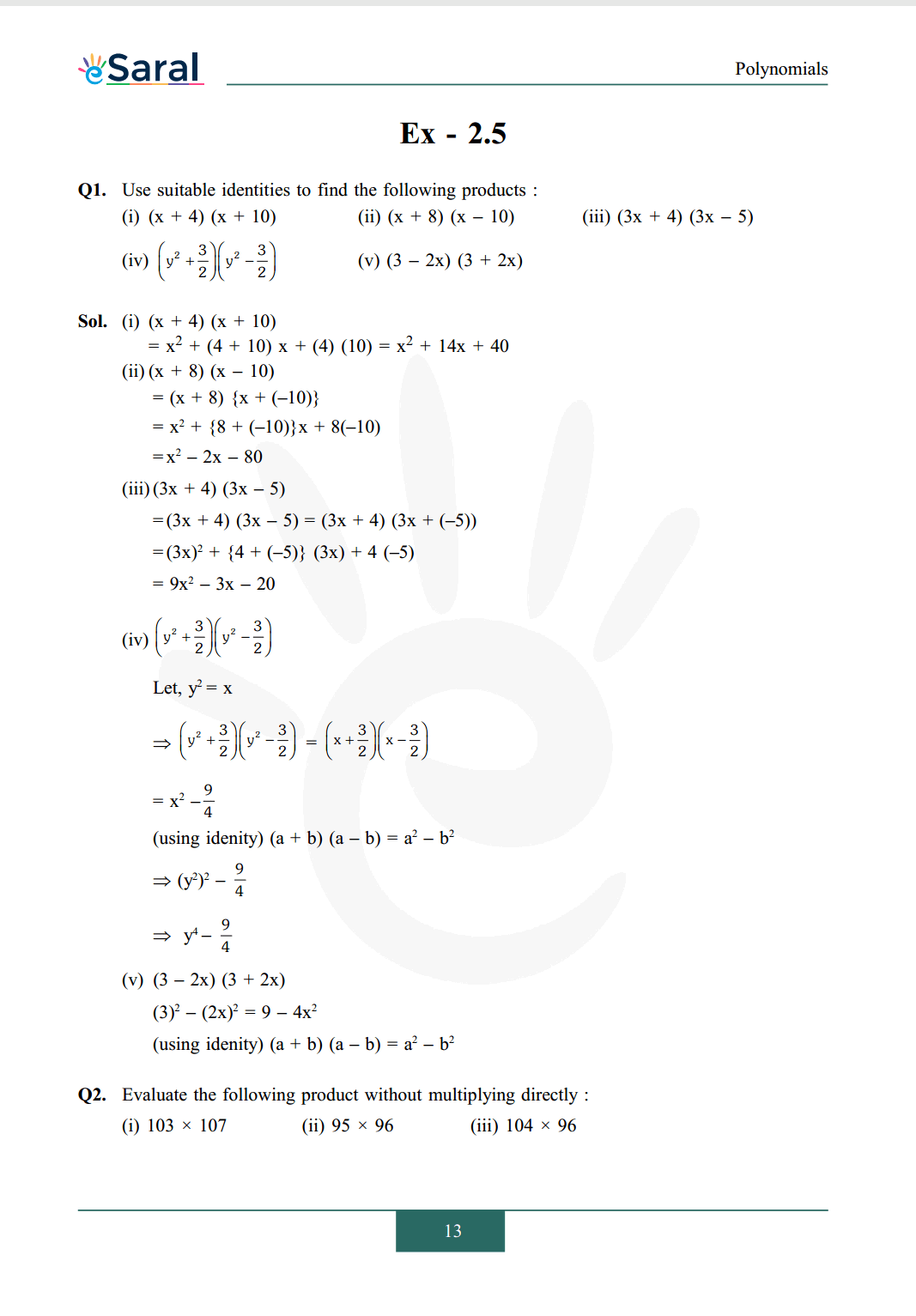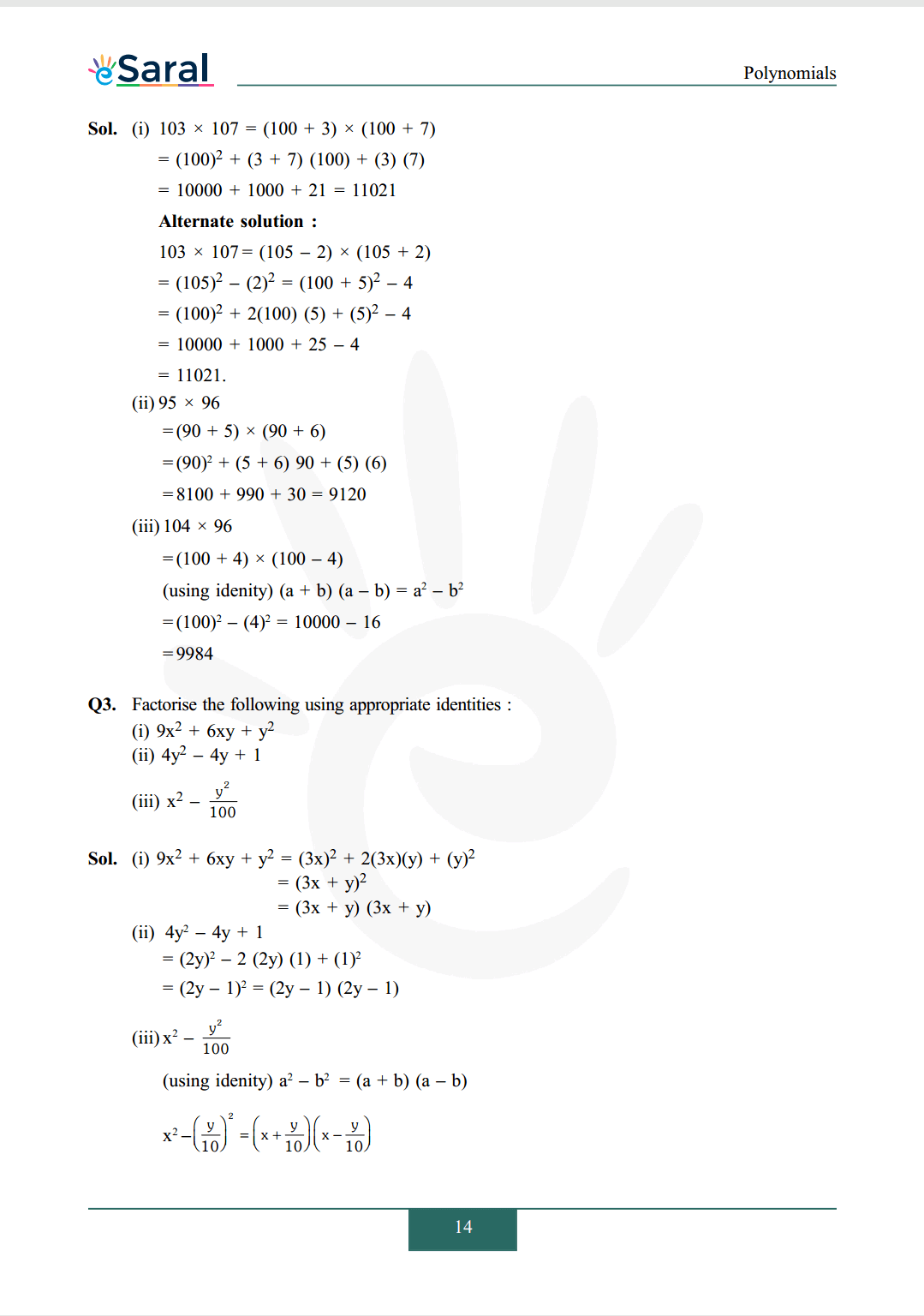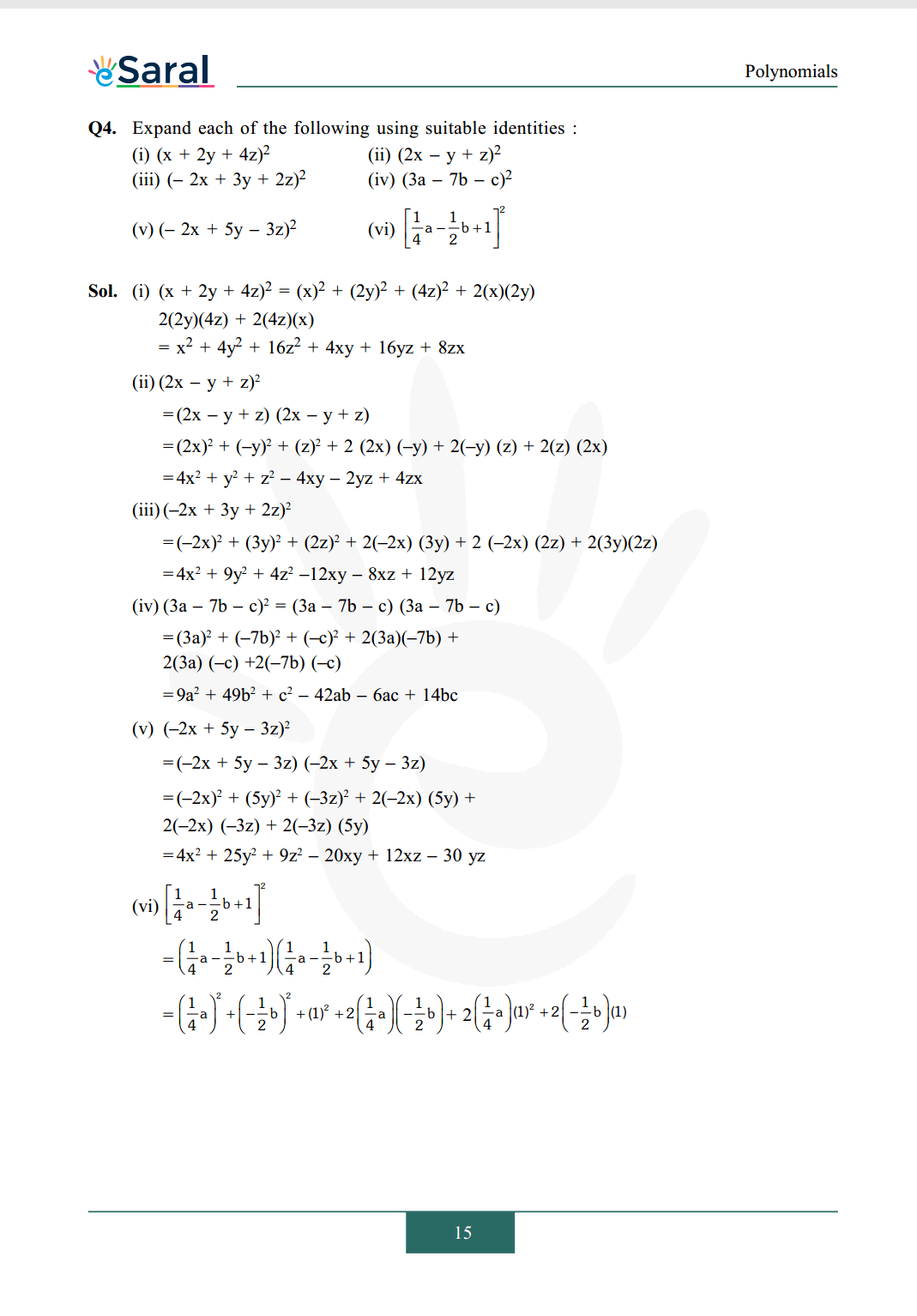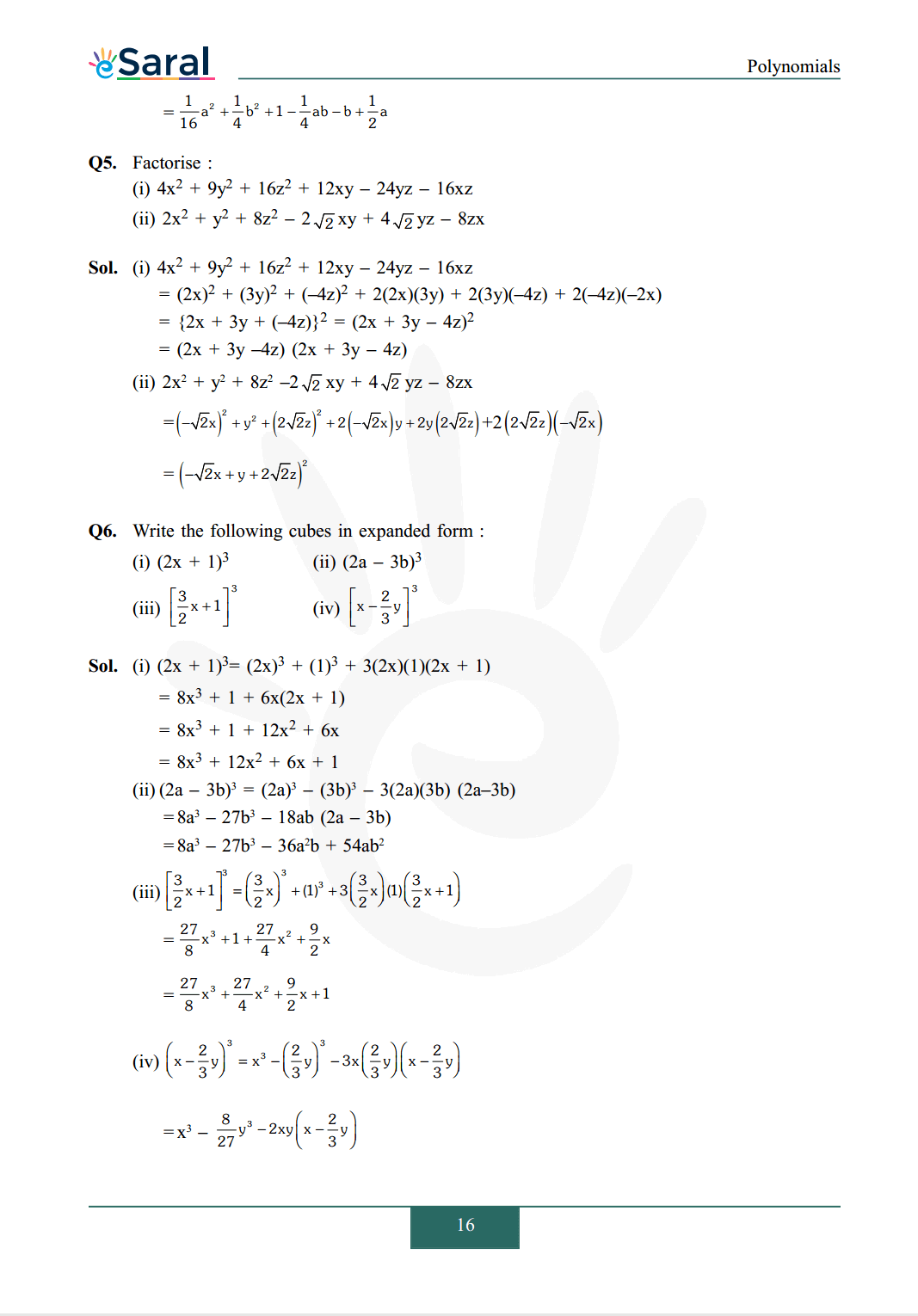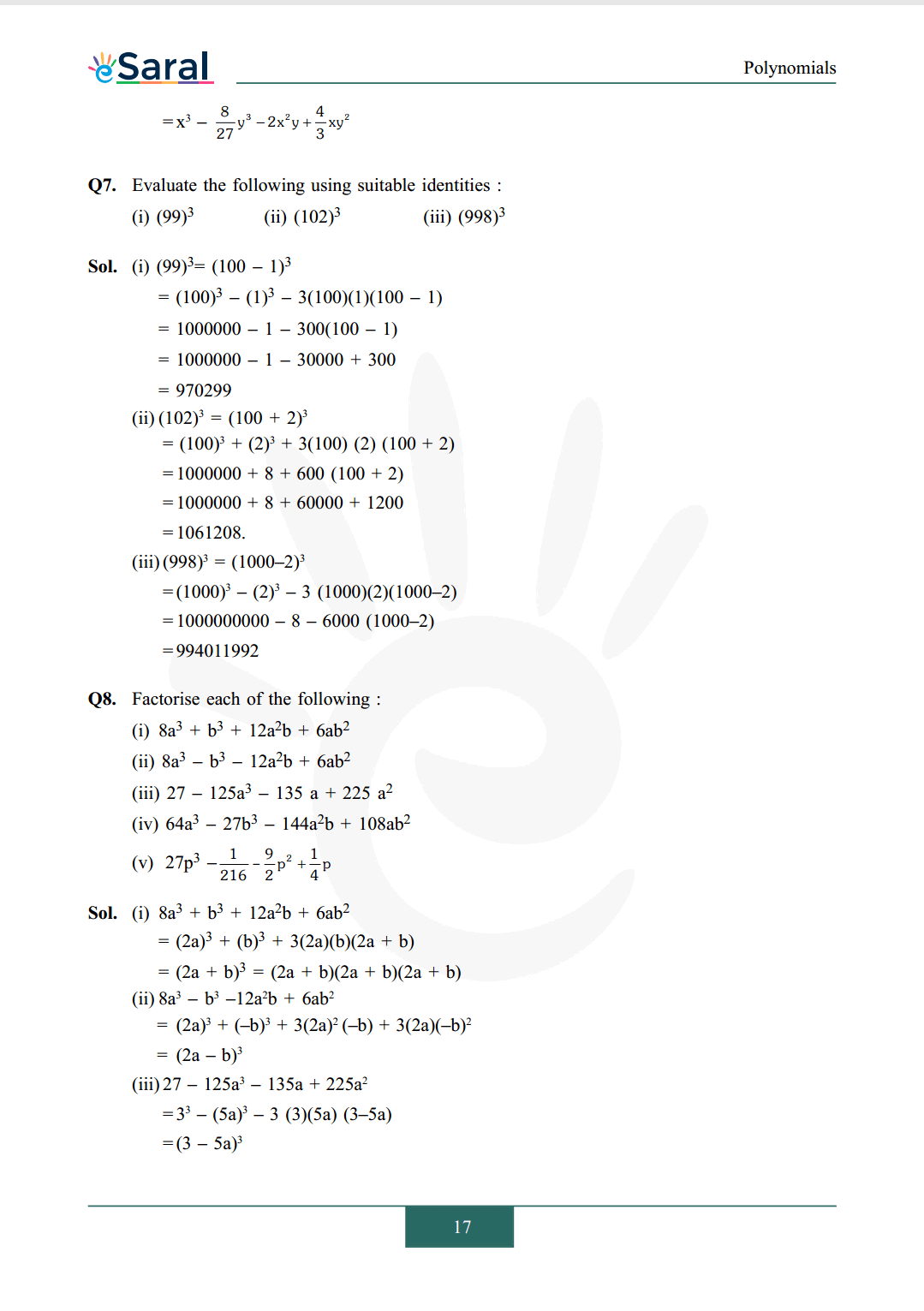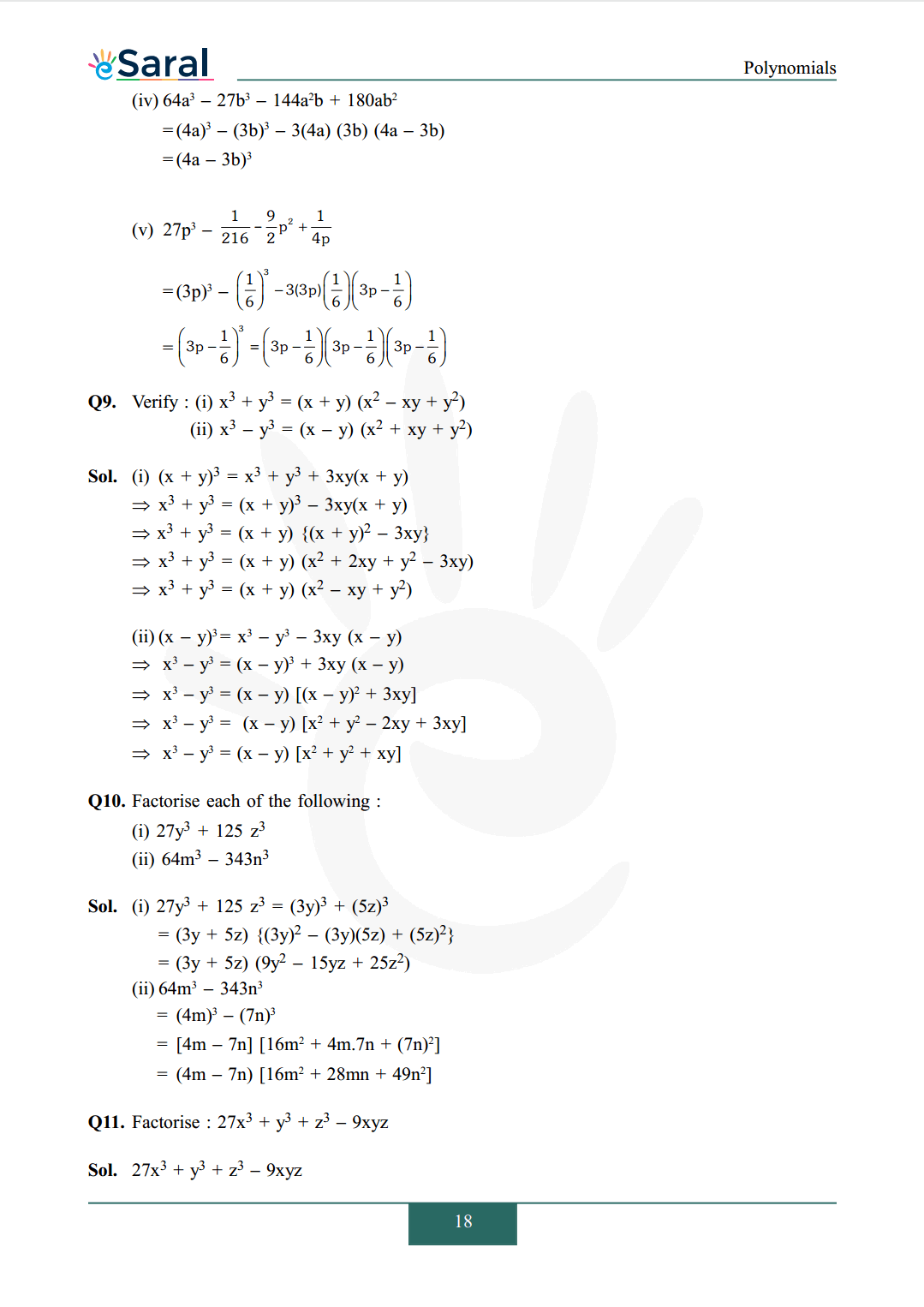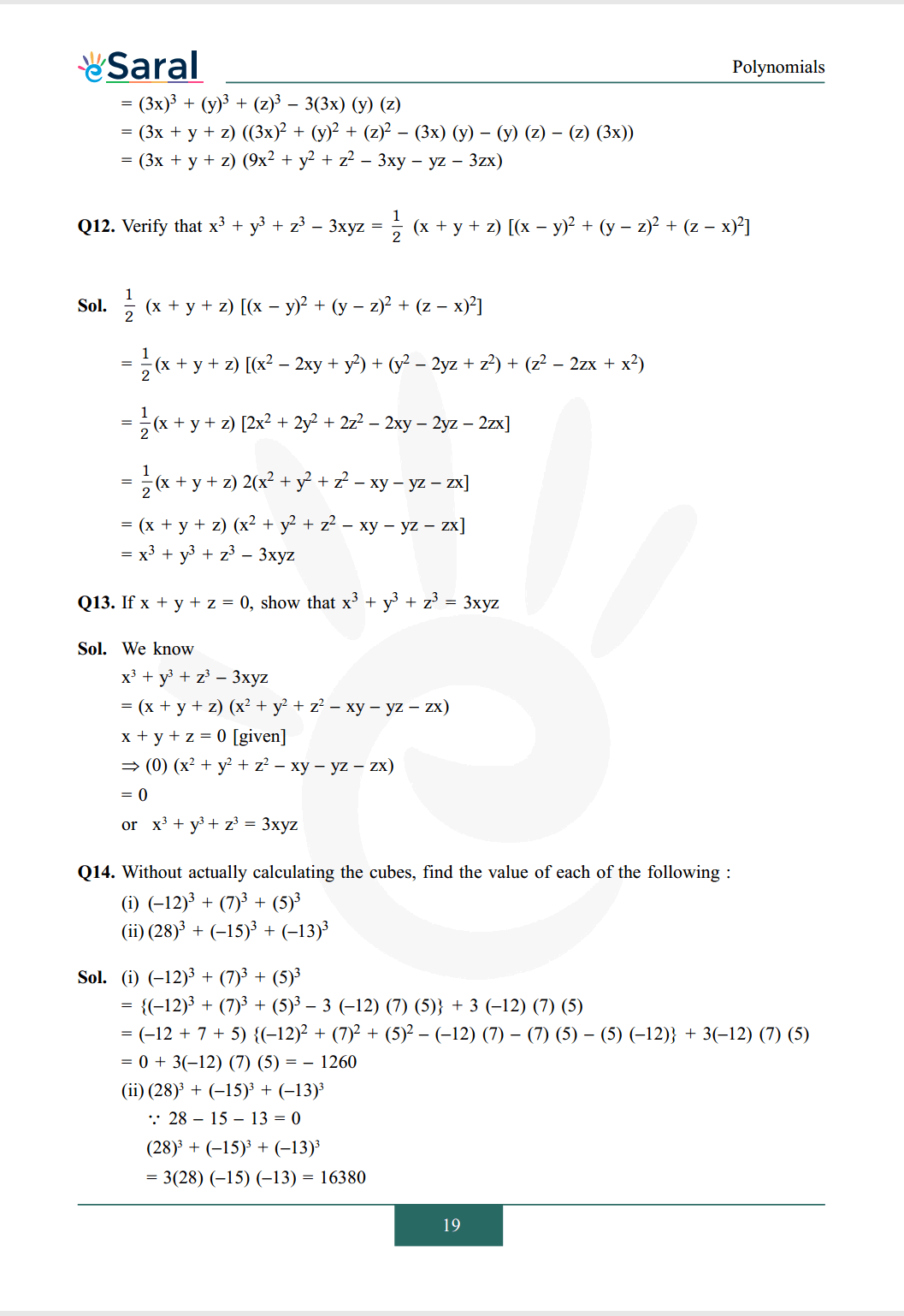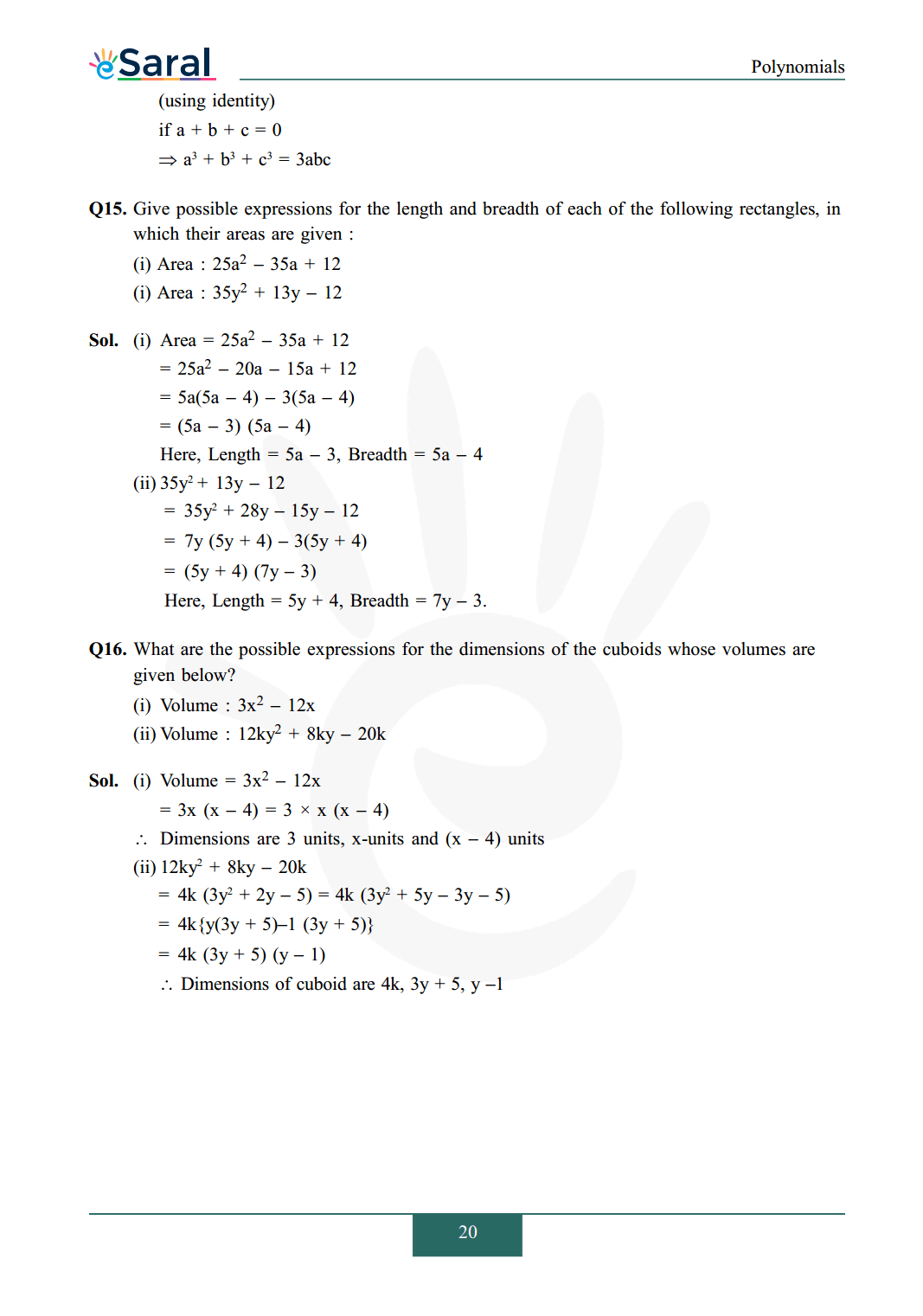#### All Questions of Chapter 2 Exercise 2.5

Once you complete the chapter 2 then you can revise Ex. 2.5 by solving following questions

Q1. Use suitable identities to find the following products :
(i) $(x+4)(x+10)$
(ii) $(\mathrm{x}+8)(\mathrm{x}-10)$
(iii) $(3 x+4)(3 x-5)$
(iv) $\left(y^{2}+\frac{3}{2}\right)\left(y^{2}-\frac{3}{2}\right)$
(v) $(3-2 x)(3+2 x)$

Q2. Evaluate the following product without multiplying directly :
(i) $103 \times 107$
(ii) $95 \times 96$
(iii) $104 \times 96$

Q3. Factorise the following using appropriate identities :
(i) $9 x^{2}+6 x y+y^{2}$
(ii) $4 \mathrm{y}^{2}-4 \mathrm{y}+1$
(iii) $\mathrm{x}^{2}-\frac{\mathrm{y}^{2}}{100}$

Q4. Expand each of the following using suitable identities :
(i) $(x+2 y+4 z)^{2}$
(ii) $(2 \mathrm{x}-\mathrm{y}+\mathrm{z})^{2}$
(iii) $(-2 x+3 y+2 z)^{2}$
(iv) $(3 a-7 b-c)^{2}$
(v) $(-2 x+5 y-3 z)^{2}$
(vi) $\left[\frac{1}{4} \mathrm{a}-\frac{1}{2} \mathrm{~b}+1\right]^{2}$

Q5. Factorise :
(i) $4 x^{2}+9 y^{2}+16 z^{2}+12 x y$
$-24 y z-16 x z$
(ii) $2 \mathrm{x}^{2}+\mathrm{y}^{2}+8 \mathrm{z}^{2}-2 \sqrt{2} \mathrm{xy}$
$+4 \sqrt{2} \mathrm{yz}-8 \mathrm{zx}$

Q6. Write the following cubes in expanded form :
(i) $(2 x+1)^{3}$
(ii) $(2 a-3 b)^{3}$
(iii) $\left[\frac{3}{2} x+1\right]^{3}$
(iv) $\left[x-\frac{2}{3} y\right]^{3}$

Q7. Evaluate the following using suitable identities :
(i) $(99)^{3}$
(ii) $(102)^{3}$
(iii) $(998)^{3}$

Q8. Factorise each of the following :
(i) $8 \mathrm{a}^{3}+\mathrm{b}^{3}+12 \mathrm{a}^{2} \mathrm{~b}+6 \mathrm{ab}^{2}$

(ii) $8 a^{3}-b^{3}-12 a^{2} b+6 a b^{2}$
(iii) $27-125 a^{3}-135 a+225 a^{2}$
(iv) $64 \mathrm{a}^{3}-27 \mathrm{~b}^{3}-144 \mathrm{a}^{2} \mathrm{~b}+108 \mathrm{ab}^{2}$
(v) $27 \mathrm{p}^{3}-\frac{1}{216}-\frac{9}{2} \mathrm{p}^{2}+\frac{1}{4} \mathrm{p}$

Q9. Verify:
(i) $x^{3}+y^{3}=(x+y)\left(x^{2}-x y+y^{2}\right)$
(ii) $x^{3}-y^{3}=(x-y)\left(x^{2}+x y+y^{2}\right)$

Q10. Factorise each of the following :
(i) $27 \mathrm{y}^{3}+125 \mathrm{z}^{3}$
(ii) $64 \mathrm{~m}^{3}-343 \mathrm{n}^{3}$

Q11. Factorise : $27 \mathrm{x}^{3}+\mathrm{y}^{3}+\mathrm{z}^{3}-9 \mathrm{xyz}$

Q12. Verify that $x^{3}+y^{3}+z^{3}-3 x y z$
$=\frac{1}{2}(\mathrm{x}+\mathrm{y}+\mathrm{z})\left[(\mathrm{x}-\mathrm{y})^{2}\right.$
$\left.+(y-z)^{2}+(z-x)^{2}\right]$

QQ13. If $x+y+z=0$, show that
$x^{3}+y^{3}+z^{3}=3 x y z$

Q14. Without actually calculating the cubes, find the value of each of the following :
(i) $(-12)^{3}+(7)^{3}+(5)^{3}$
(ii) $(28)^{3}+(-15)^{3}+(-13)^{3}$

Q15. Give possible expressions for the length and breadth of each of the following rectangles, in which their areas are given :
(i) Area : $25 \mathrm{a}^{2}-35 \mathrm{a}+12$
(i) Area : $35 \mathrm{y}^{2}+13 \mathrm{y}-12$

Q16. What are the possible expressions for the dimensions of the cuboids whose volumes are given below?
(i) Volume : $3 \mathrm{x}^{2}-12 \mathrm{x}$
(ii) Volume : $12 \mathrm{ky}^{2}+8 \mathrm{ky}-20 \mathrm{k}$

Class 9 NCERT Maths Book PDF

Class 9 NCERT Maths Exemplar PDF

Class 9 Maths Chapter 1 Exercise 1.1 Solutions Free

Class 9 Maths Chapter 1 Exercise 1.2 Solutions Free

Class 9 Maths Chapter 1 Exercise 1.3 Solutions Free

Class 9 Maths Chapter 1 Exercise 1.4 Solutions Free

Class 9 Maths Chapter 1 Exercise 1.5 Solutions Free

Class 9 Maths Chapter 1 Exercise 1.6 Solutions Free

If you have any Confusion related to NCERT Solutions for Class 9 Maths chapter 2 Exercise 2.5 then feel free to ask in the comments section down below.

To watch Free Learning Videos on Class 9 by Kota’s top Faculties Install the eSaral App